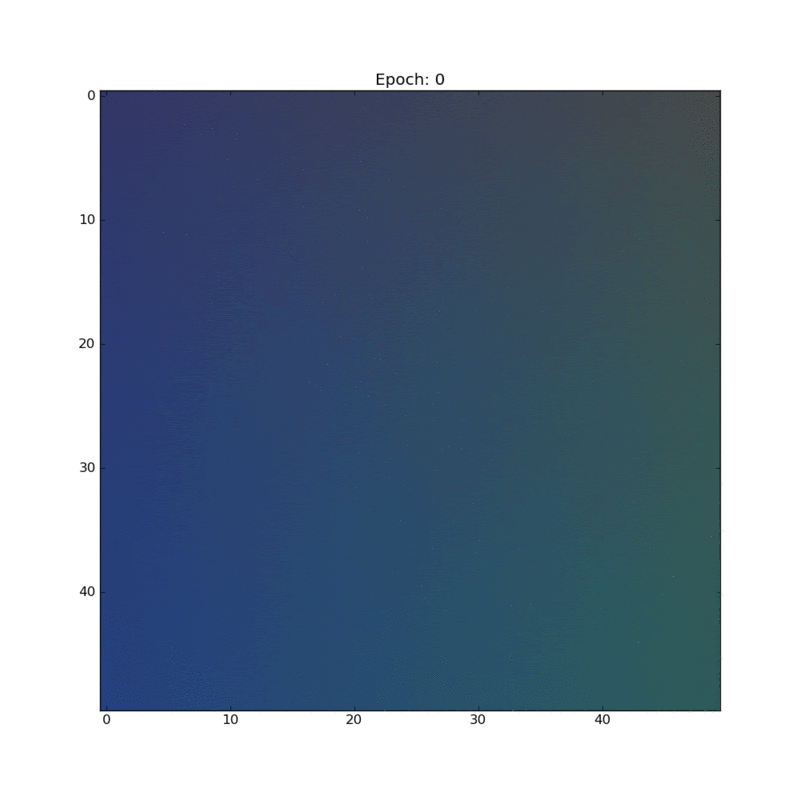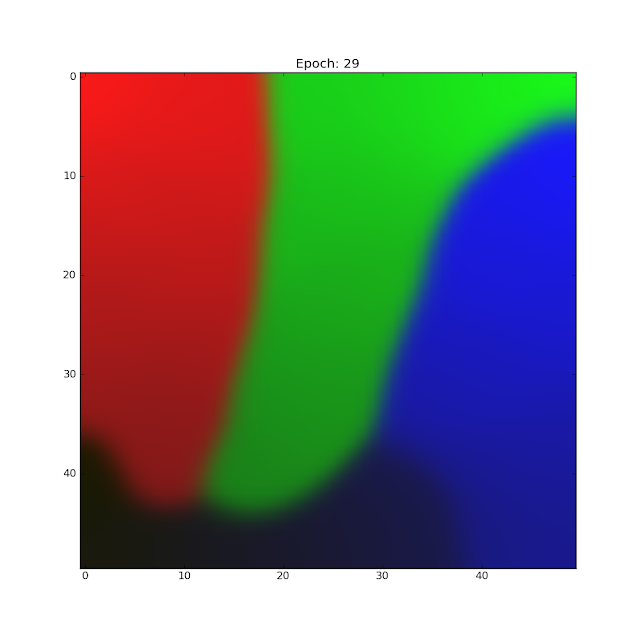## Wednesday, 30 March 2016

### Unsupervised Learning: Self Organizing Maps

Most machine learning techniques like logistic regression, linear regression, and neural networks do something that is called supervised learning. This means that we need to supply examples of "correct" data to the machine learning algorithm for it to learn the patterns in the data. The goal of unsupervised learning is to automatically find clusters of similar data in a huge unlabeled set of data. Humans can do this easily with some types of data. If you saw a 2D scatter plot of points you'd easily be able to identify clusters in the data by visual examination. I've always considered unsupervised learning cooler than supervised mostly because it feels like that's what a general AI should be able to do.

Self organizing maps (SOMs) are one technique used to find these clusters in the data. A self organizing map looks a little similar to a neural network at first. But the kind of operation that it does is very different. An SOM has a lattice of nodes. This lattice is usually one dimensional(arranged in a linear array) or 2 dimensional (arranged in a matrix). As the self organizing map is trained, the lattice of will be partitioned into separate classes. Since one of the main uses of self organizing maps is visualizing higher dimensional data, the lattice is is rarely more than two dimensions wide. The clusters in the higher dimensional data can be visualized on the 2D lattice.  Associated with each of the nodes in the lattice is a weight vector that has the same dimension as the input data vectors. To train the self organizing map we take each element in the data set and find the node with the weight that's closest to the element (Using the euclidean distance as the measure of closeness. Other measures can be used too.). Then we define a neighbourhood of nodes that are 'close' to this best matching unit and we adjust the weights of this entire neighbourhood of weights to be a little closer to the value of the element from the dataset. How much the weights are adjusted depends on the neighbour falloff function and on the learning rate. This can be expressed in one simple equation.
$$W_{uv}(t+1) = W_{uv}(t) + \Omega (u,v,t) \alpha (t)[x_n - W_{uv}(t)]$$
In each iteration, the learning rate and the size of the neighbourhood function is reduced by a small amount. The neighbourhood size is initially set to a large value. This allows the algorithm to initially affect the weights on a global scale and then over time adjust smaller and smaller regions of the map to train it.

In my implementation I used a Gaussian neighbourhood function whose variance decayed exponentially. The learning rate started off at 0.1 and decayed exponentially from there.

To check my implementation, I tested something that is a common use for SOMs. Clustering colors in an RGB color space. Colors are easy to visualize and I thought it would make a good demo. I first generated a random data set of bluish, reddish, greenish and dark bluish colors.A random sampling of 4 different colors for the dataset.
I initialized the weights randomly and started the training. For this particular experiment I used a 2D 50x50 lattice of nodes. Since each node has a weight that is a 3 dimensional vector that represents a color, we can visualize the SOM node lattice as an RGB image. Stacking together the weight visualization after each epoch (One pass through the training set) of training gave me this really beautiful looking animation! :DAnimation that shows the self organizing map after each epoch of training.
You can clearly see the different clusters of color slowly resolving as the training happens. The initial changes are more global due to the large neighbourhood. But as the training progresses the adjustments to the weights become more and more local. There is also a very nice separation of the different colors that were present in the first image. You can see that the brighter colors seem to be congregating towards the top of the image and the darker colors towards the bottom. The darkest blue (maybe even a little black) ended up in the bottom left corner surrounded by the darker hues of red, green and blue.The final map after 30 epochs of training.